# RD Sharma Solutions For Class 7 Maths Exercise 3.1 Chapter 3 Decimals

The main objective of providing exercise-wise solutions in PDF is to help students speed up their exam preparation. The solutions are designed based on the latest CBSE syllabus and exam pattern. Understanding the concepts before solving the exercise wise problems is very important to obtain a better score in the exam. The questions present in this have been solved by BYJU’S experts in Maths, and these solutions guide students to study well and solve lengthy problems in a shorter duration. The students can download solutions which are available in PDF format easily. RD Sharma Solutions for Class 6 Maths Chapter 3 Decimals Exercise 3.1 are provided here. By practising the RD Sharma Solutions for Class 7, students will be able to score high in exams.

## Download the PDF of RD Sharma Solutions For Class 7 Maths Chapter 3 – Decimals Exercise 3.1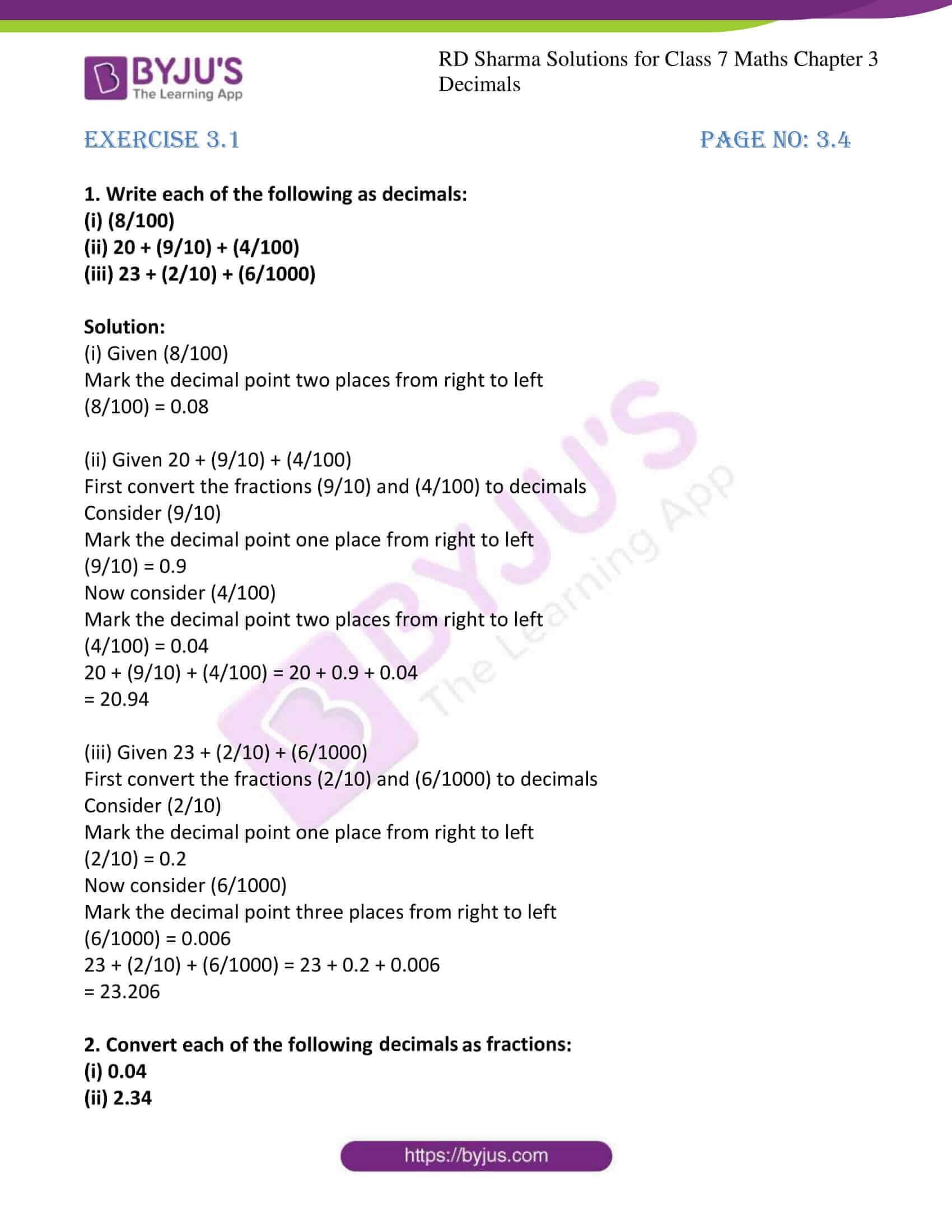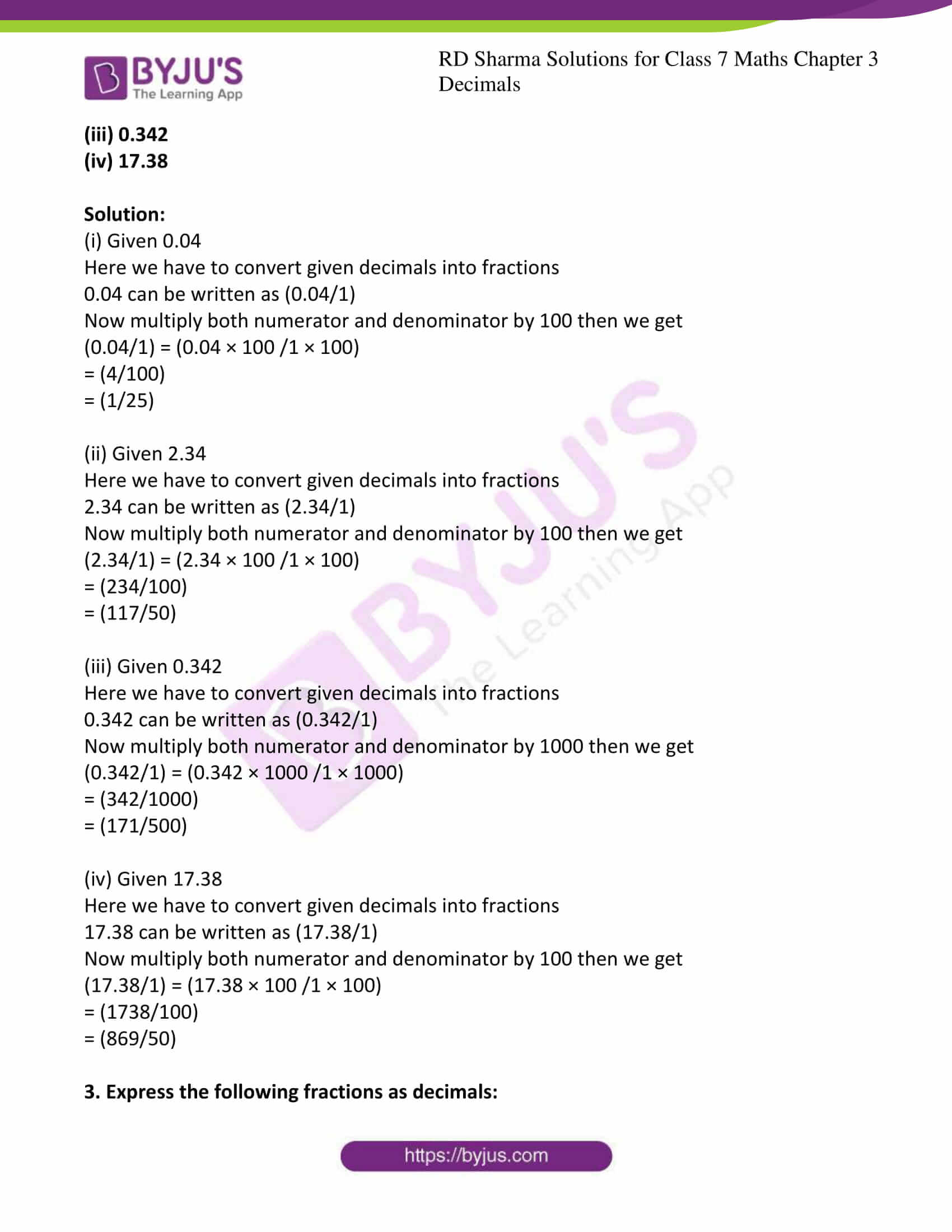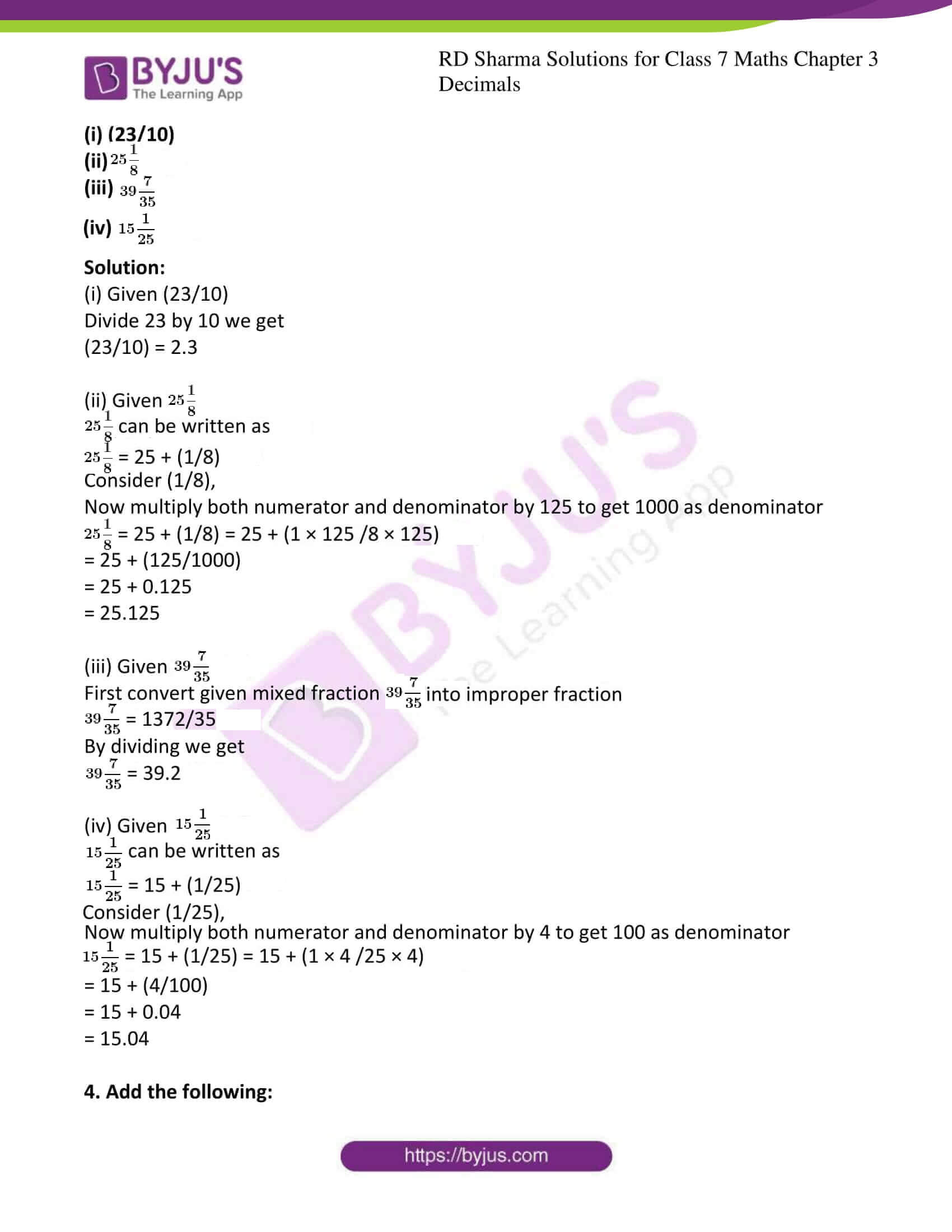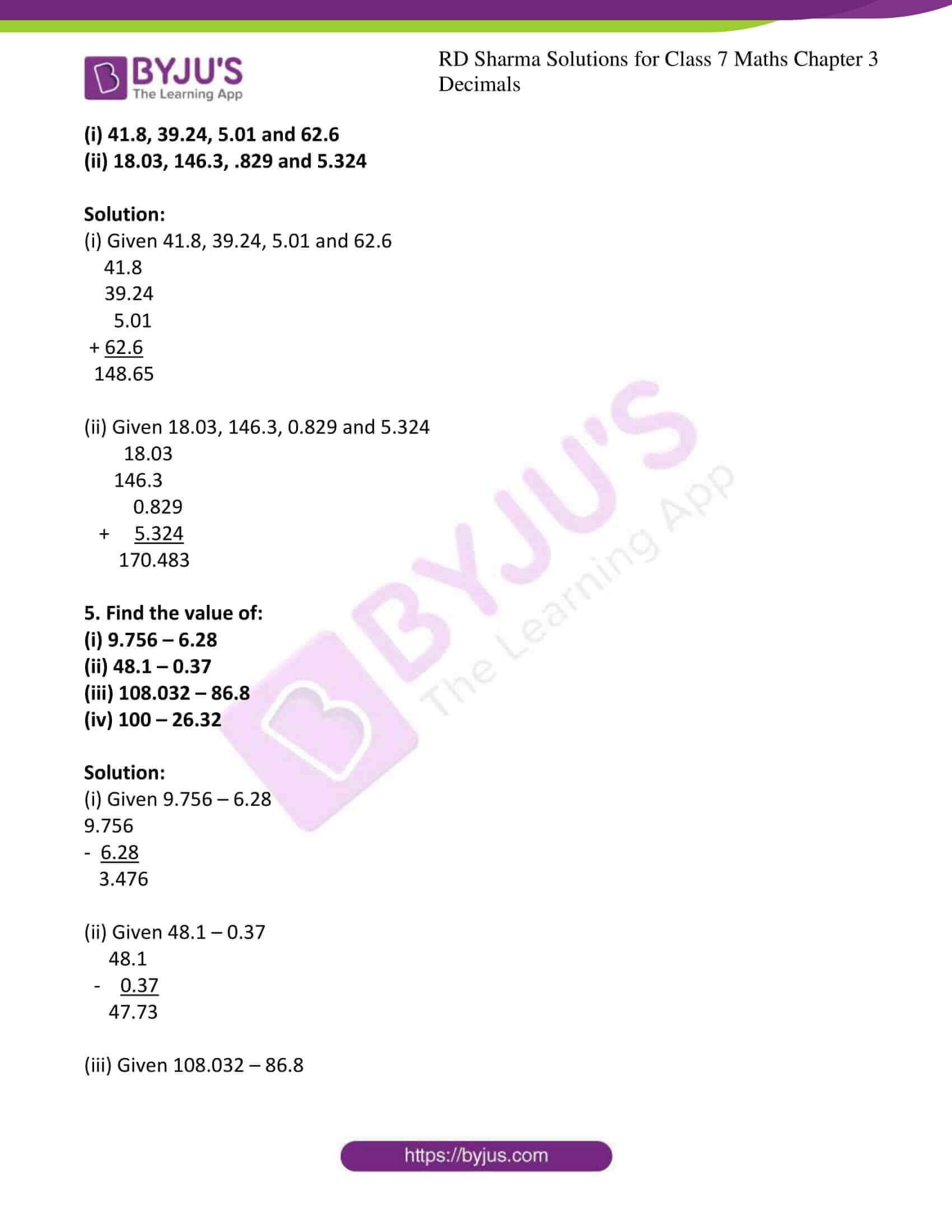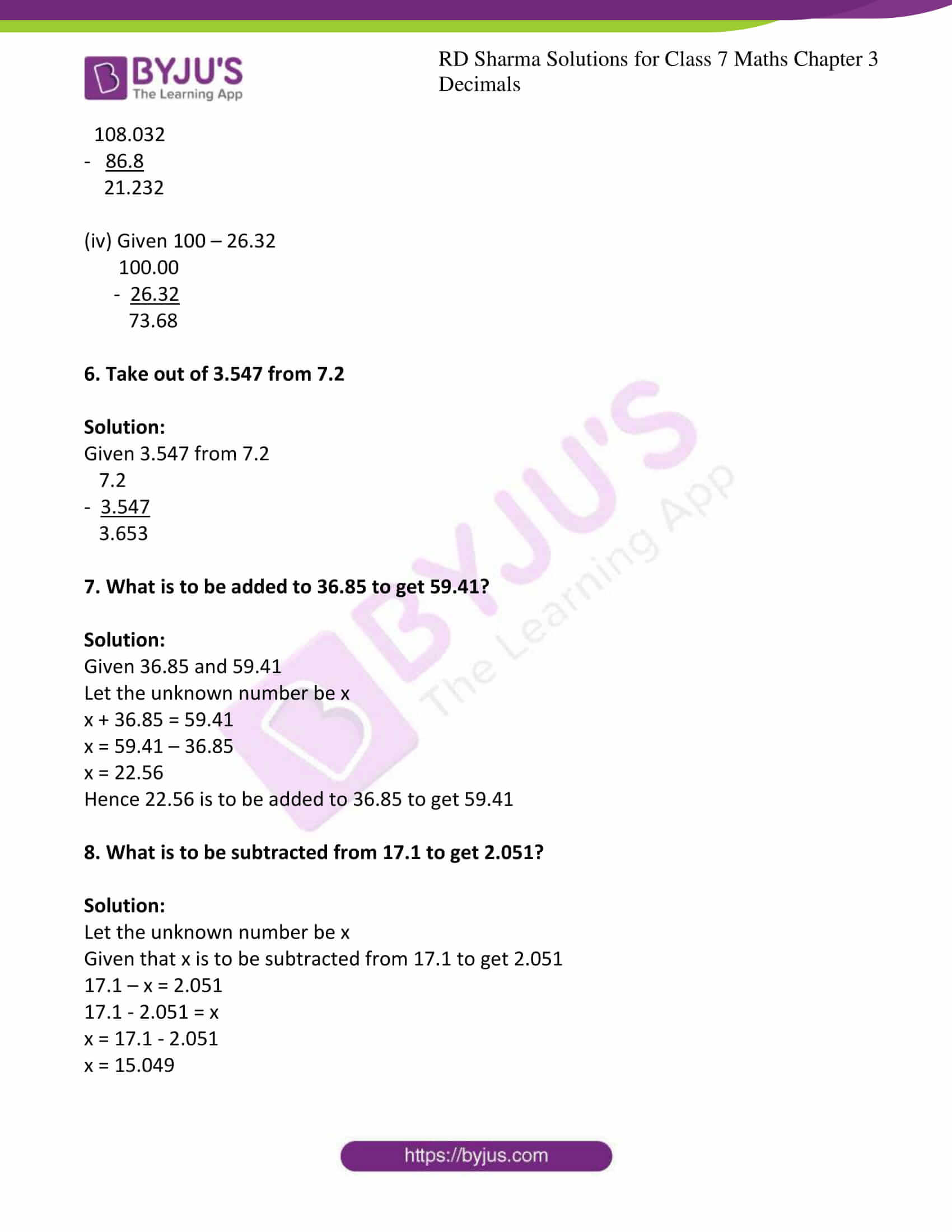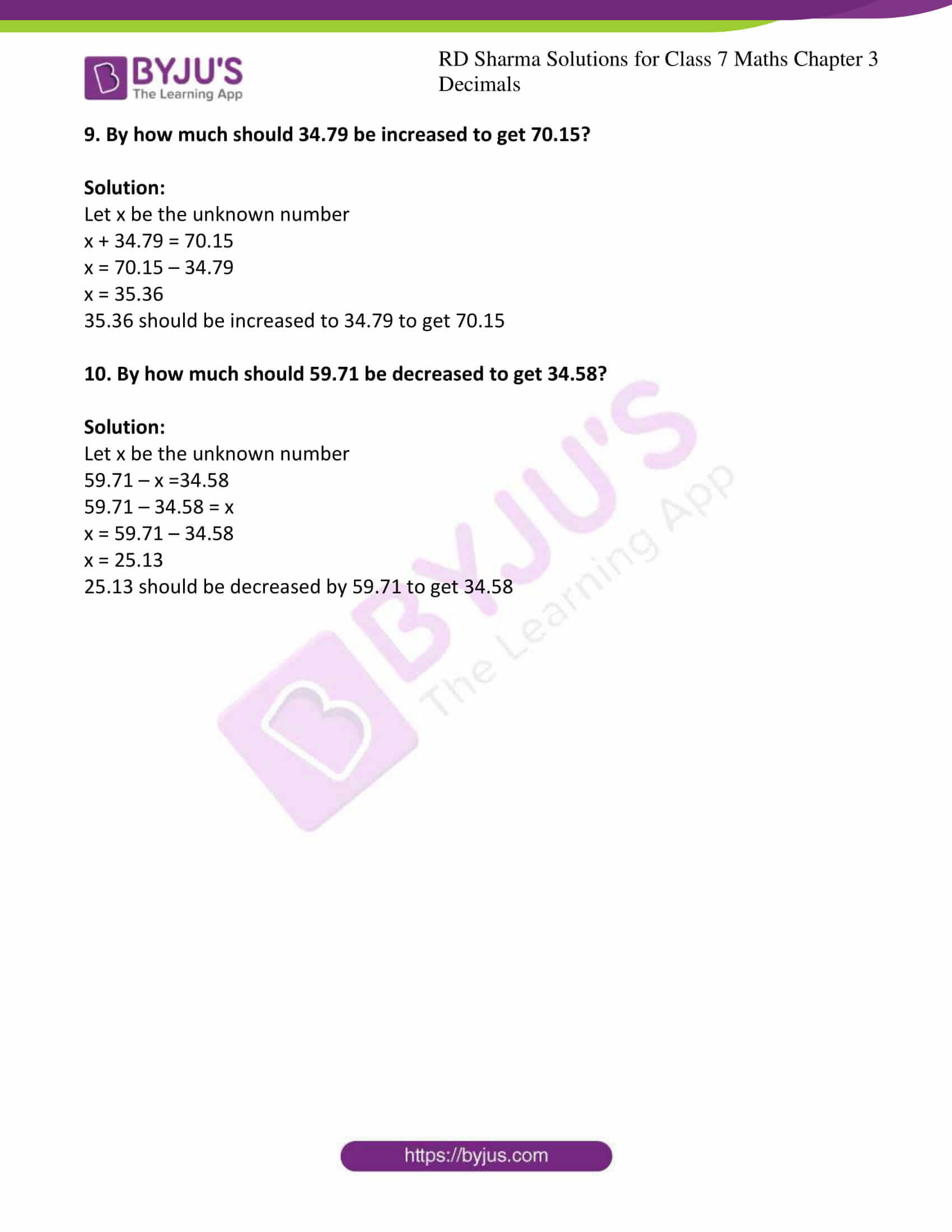### Access answers to Maths RD Sharma Solutions For Class 7 Chapter 3 – Decimals Exercise 3.1

1. Write each of the following as decimals:

(i) (8/100)

(ii) 20 + (9/10) + (4/100)

(iii) 23 + (2/10) + (6/1000)

Solution:

(i) Given (8/100)

Mark the decimal point two places from right to left

(8/100) = 0.08

(ii) Given 20 + (9/10) + (4/100)

First convert the fractions (9/10) and (4/100) to decimals

Consider (9/10)

Mark the decimal point one place from right to left

(9/10) = 0.9

Now consider (4/100)

Mark the decimal point two places from right to left

(4/100) = 0.04

20 + (9/10) + (4/100) = 20 + 0.9 + 0.04

= 20.94

(iii) Given 23 + (2/10) + (6/1000)

First convert the fractions (2/10) and (6/1000) to decimals

Consider (2/10)

Mark the decimal point one place from right to left

(2/10) = 0.2

Now consider (6/1000)

Mark the decimal point three places from right to left

(6/1000) = 0.006

23 + (2/10) + (6/1000) = 23 + 0.2 + 0.006

= 23.206

2. Convert each of the following decimals as fractions:

(i) 0.04

(ii) 2.34

(iii) 0.342

(iv) 17.38

Solution:

(i) Given 0.04

Here we have to convert given decimals into fractions

0.04 can be written as (0.04/1)

Now multiply both numerator and denominator by 100 then we get

(0.04/1) = (0.04 × 100 /1 × 100)

= (4/100)

= (1/25)

(ii) Given 2.34

Here we have to convert given decimals into fractions

2.34 can be written as (2.34/1)

Now multiply both numerator and denominator by 100 then we get

(2.34/1) = (2.34 × 100 /1 × 100)

= (234/100)

= (117/50)

(iii) Given 0.342

Here we have to convert given decimals into fractions

0.342 can be written as (0.342/1)

Now multiply both numerator and denominator by 1000 then we get

(0.342/1) = (0.342 × 1000 /1 × 1000)

= (342/1000)

= (171/500)

(iv) Given 17.38

Here we have to convert given decimals into fractions

17.38 can be written as (17.38/1)

Now multiply both numerator and denominator by 100 then we get

(17.38/1) = (17.38 × 100 /1 × 100)

= (1738/100)

= (869/50)

3. Express the following fractions as decimals:

(i) (23/10)

(ii) $$25\frac{1}{8}$$

(iii) $$39\frac{7}{35}$$

(iv) $$15\frac{1}{25}$$

Solution:

(i) Given (23/10)

Divide 23 by 10 we get

(23/10) = 2.3

(ii) Given $$25\frac{1}{8}$$

$$25\frac{1}{8}$$ can be written as

$$25\frac{1}{8}$$ = 25 + (1/8)

Consider (1/8),

Now multiply both numerator and denominator by 125 to get 1000 as denominator

$$25\frac{1}{8}$$= 25 + (1/8) = 25 + (1 × 125 /8 × 125)

= 25 + (125/1000)

= 25 + 0.125

= 25.125

(iii) Given $$39\frac{7}{35}$$

First convert given mixed fraction $$39\frac{7}{35}$$ into improper fraction

$$39\frac{7}{35}$$ = 1372/35

By dividing we get

$$39\frac{7}{35}$$ = 39.2

(iv) Given $$15\frac{1}{25}$$

$$15\frac{1}{25}$$ can be written as

$$15\frac{1}{25}$$ = 15 + (1/25)

Consider (1/25),

Now multiply both numerator and denominator by 4 to get 100 as denominator

$$15\frac{1}{25}$$ = 15 + (1/25) = 15 + (1 × 4 /25 × 4)

= 15 + (4/100)

= 15 + 0.04

= 15.04

(i) 41.8, 39.24, 5.01 and 62.6

(ii) 18.03, 146.3, .829 and 5.324

Solution:

(i) Given 41.8, 39.24, 5.01 and 62.6

41.8

39.24

5.01

+ 62.6

148.65

(ii) Given 18.03, 146.3, 0.829 and 5.324

18.03

146.3

0.829

+ 5.324

170.483

5. Find the value of:

(i) 9.756 – 6.28

(ii) 48.1 – 0.37

(iii) 108.032 – 86.8

(iv) 100 – 26.32

Solution:

(i) Given 9.756 – 6.28

9.756

– 6.28

3.476

(ii) Given 48.1 – 0.37

48.1

– 0.37

47.73

(iii) Given 108.032 – 86.8

108.032

– 86.8

21.232

(iv) Given 100 – 26.32

100.00

– 26.32

73.68

6. Take out of 3.547 from 7.2

Solution:

Given 3.547 from 7.2

7.2

– 3.547

3.653

7. What is to be added to 36.85 to get 59.41?

Solution:

Given 36.85 and 59.41

Let the unknown number be x

x + 36.85 = 59.41

x = 59.41 – 36.85

x = 22.56

Hence 22.56 is to be added to 36.85 to get 59.41

8. What is to be subtracted from 17.1 to get 2.051?

Solution:

Let the unknown number be x

Given that x is to be subtracted from 17.1 to get 2.051

17.1 – x = 2.051

17.1 – 2.051 = x

x = 17.1 – 2.051

x = 15.049

9. By how much should 34.79 be increased to get 70.15?

Solution:

Let x be the unknown number

x + 34.79 = 70.15

x = 70.15 – 34.79

x = 35.36

35.36 should be increased to 34.79 to get 70.15

10. By how much should 59.71 be decreased to get 34.58?

Solution:

Let x be the unknown number

59.71 – x =34.58

59.71 – 34.58 = x

x = 59.71 – 34.58

x = 25.13

25.13 should be decreased by 59.71 to get 34.58

### RD Sharma Solutions for Class 7 Maths Chapter 3 – Decimals Exercise 3.1

RD Sharma Solutions for Class 7 Maths Chapter 3 Decimals Exercise 3.1 has problems which are based on multiplication and division of decimals. Some of the topics focused prior to Exercise 3.1 are listed below.

• Definition of decimals
• Types of decimals
• Comparing decimals
• Addition and subtraction of decimals